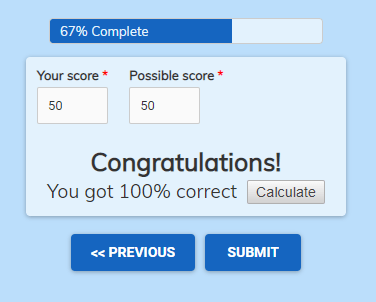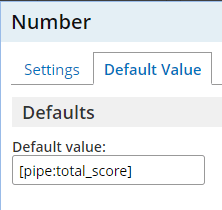# Show Percentages of Calculated AmountsThe Calculations feature lets forms process basic equations and makes quiz forms easy. Mostly used with the Scoring feature, calculations let form owners set values for form answers then use those answers in equations. Form owners can show percentages using calculated values if needed.

The most common use for Scoring is with an online quiz. The form owner starts by setting values for each answer, then the form tracks the scoring total. At the end of the form, the result displays as the total score and the total possible.

The built-in Piping codes show the scoring totals on the form, but displaying as percentages requires an extra step.

## Show Percentages

Finding the percentage of a value follows the basic format:

`(Value / Max value) * 100`

Using that equation with the value of 5 and max value of 20:

`(5/20)*100 = (0.25)*100 = 25`

Appending the calculated value with the percent sign (%) displays the percentage as ‘25%’.

## Practical UseQuizzes:
For use with a quiz form, the form owner places Number items on the last page of the form with the pipe codes as the default values. These fields provide the values for the Calculation item equation:

`(id33/id34)*100`

Orders:
Use the Scoring features with orders to calculate custom values. These values can show other items with Rules, such as discounts or extra options. See more about auto-discounts at a previous article.